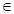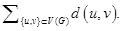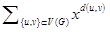Archive
Special Issues
The Wiener Index and the Hosoya Polynomial of the Jahangir Graphs
Applied and Computational Mathematics
Volume 5, Issue 3, June 2016, Pages: 138-141
Received: Apr. 21, 2016; Accepted: May 3, 2016; Published: Jul. 13, 2016
Authors
Shaohui Wang, Department of Mathematics, University of Mississippi, University, MS, USA; Department of Mathematics and Computer Science, Adelphi University, Garden City, NY, USA
Mohammad Reza Farahani, Department of Applied Mathematics, Iran University of Science and Technology (IUST) Narmak, Tehran, Iran
M. R. Rajesh Kanna, Department of Mathematics, Maharani's Science College for Women, Mysore, India
Muhammad Kamran Jamil, Department of Mathematics, Riphah Institute of Computing and Applied Sciences (RICAS), Riphah International University, Lahore, Pakistan
R. Pradeep Kumar, Department of Mathematics, the National Institute of Engineering, Mysuru, India
Article ToolsAbstractPDF (424KB)HTML
Abstract
Let G be a simple connected graph having vertex set V and edge set E. The vertex-set and edge-set of G denoted by V(G) and E(G), respectively. The length of the smallest path between vertices u,vV(G) is called the distance, d(u,v), between the vertices u,v. Mathematical chemistry is the area of research engaged in new application of mathematics in chemistry. In mathematics chemistry, we have many topological indices for any molecular graph, that they are invariant on the graph automorphism. In this research paper, we computing the Wiener index and the Hosoya polynomial of the Jahangir graphs J 5,m for all integer number m ≥3. The Wiener index is the sum of distances between all pairs of vertices of G as W(G)=And the Hosoya polynomial of G is H(G,x)=, where d(u,v) denotes the distance between vertices u and v.
Keywords
Regular Graphs, Connected Graphs, Jahangir Graphs, Topological Indices, Hosoya Polynomial, Wiener Index, Distances
Shaohui Wang, Mohammad Reza Farahani, M. R. Rajesh Kanna, Muhammad Kamran Jamil, R. Pradeep Kumar, The Wiener Index and the Hosoya Polynomial of the Jahangir Graphs, Applied and Computational Mathematics. Vol. 5, No. 3, 2016, pp. 138-141. doi: 10.11648/j.acm.20160503.17
References

H. Wiener, Structural determination of paraffin boiling points, J. Amer. Chem. Soc. 69 (1947), 17–20.

P. V. Khadikar and S. Karmarkar. On the estimation of PI index of polyacenes. Acta Chim. Slov. 49, 2002, 755-771.

H. Hosoya. On some counting polynomials in chemistry. Discrete Appl. Math. 19, (1988), 239-257.

Gutman, O. E. Polansky, Mathematical Concepts in Organic Chemistry Springer, Berlin, 1986.

Gutman, S. Klavžar, B. Mohar. Fifty years of the Wiener index, (Eds.), MATCH Commun. Math. Comput. Chem. 35, 1-259 (1997).

Gutman, S. Klavžar, B. Mohar. Fiftieth anniversary of the Wiener index, (Eds.), Discrete Appl. Math. 80, 1--113 (1997).

E. Estrada, O. Ivanciuc, I. Gutman, A. Gutierrez and L. Rodrguez, Extended Wiener Indices. A New Set of Descriptors for Quantitative Structure Property Studies, New J. Chem. 22 (1998) 819-823.

H. Hosoya, A newly proposed quantity characterizing the topological nature of structural isomers of saturated hydrocarbons, Bull. Chem. Soc. Japan 44 (1971), 2332-2339.

Gutman, S. Klavžar, M. Petkovsek and P. Zigert, On Hosoya polynomials of benzenoid graphs, MATCH Commun. Math. Comput. Chem., 43 (2001) 49-66.

A. Dobrynin, R. Entringer, I. Gutman, Wiener index of trees: Theory and applications, Acta Appl. Math. 66 (2001), 211–249.

M. V. Diudea. Hosoya polynomial in Tori. MATCH Commun. Math. Comput. Chem. 45, (2002), 109-122.

M. Eliasi, B. Taeri, Hosoya polynomial of zigzag polyhex Nanotorus, J. Serb. Chem. Soc. 73 (2008) 313–319.

S. Xu, H. Zhang, Hosoya polynomials of armchair open–ended Nanotubes, Int. J. Quant. Chem. 107 (2007) 586–596.

J. Chen, S. Xu, H. Zhang, Hosoya polynomials of TUC4C8(R) Nanotubes, Int. J. Quant. Chem. 109 (2009) 641–649.

S. Xu, H. Zhang, The Hosoya polynomial decomposition for catacondensed benzenoid graphs, Discr. Appl. Math. 156 (2008) 2930–2938.

H. Shabani, A. R. Ashrafi, Applications of the matrix package MATLAB in computing the Wiener polynomial of armchair polyhex nanotubes and nanotori, J. Comput. Theor. Nanosci. 7 (2010) 1143–1146.

M. Knor, P. Potočnik and R. Škrekovski. Wiener index of iterated line graphs of trees homeomorphic to the claw K1;3. Ars Math. Contemp. 6 (2013), 211–219.

M. R. Farahani. Hosoya polynomial, Wiener and Hyper-Wiener indices of some regular graphs. Informatics Engineering, an International Journal (IEIJ), 1 (1), (2013), 9-13.

M. R. Farahani, M. P. Vlad. On the Schultz, Modified Schultz and the Hosoya polynomials and Derived Indices of Capra-designed planar Benzenoid. Studia UBB Chemia. 57 (4), (2012), 55-63.

M. R. Farahani. Schultz indices and Schultz Polynomials of Harary graph. Pacific Journal of Applied Mathematics. 6 (3), (2014), 77-84.

M. R. Farahani. Hosoya, Schultz, Modified Schultz Polynomials and Their Topological Indices of Benzene Molecules: First Members of Polycyclic Aromatic Hydrocarbons (PAHs). International Journal of Theoretical Chemistry. 1 (2), (2013), 09-16.

M. R. Farahani. On the Schultz polynomial, Modified Schultz polynomial, Hosoya polynomial and Wiener index of Circumcoronene series of benzenoid, J. Applied Math. & Info. 31 (5-6), (2013). 595-608.

K. Ali, E. T. Baskoro and I. Tomescu, On the Ramzey number of Paths and Jahangir graph J3,m. 3rd International Conference on 21st Century Mathematics 2007, GC University Lahoor Pakistan, March 2007.

D. A. Mojdeh and A. N. Ghameshlou. Domination in Jahangir Graph J2,m. Int. J. Contemp. Math. Sciences, 2 (24), (2007), 1193-1199.

Lourdusamy. Covering Cover Pebbling Number for Jahangir Graph J2, m. Sciencia Acta Xaveriana. 4 (2), 2012, 59-64.

Lourdusamy, S. Samuel Jeyaseelan and T. Mathivanan. On Pebbling Jahangir Graph. Gen. Math. Notes, 5 (2), 2011, 42-49.

Lourdusamy and T. Mathivanan. The 2t-Pebbling Property on the Jahangir Graph J2;m. Gen. Math. Notes, 28 (1), 2015, 18-39.

Lourdusamy and T. Mathivanan. The t-pebbling number of Jahangir graph J3,m. Proyecciones Journal of Mathematics. 34 (2), 161-174, 2015.

Lourdusamy, S. Samuel Jeyaseelan and T. Mathivanan. The t-Pebbling Number of Jahangir Graph. International J. Math. Combin. 1, (2012), 92-95.

M. Ramachandran and N. Parvathi. The Medium Domination Number of a Jahangir Graph Jm,n. Indian Journal of Science and Technology, 8 (5), 400–406, 2015

M. R. Farahani, Hosoya Polynomial and Wiener Index of Jahangir graphs J2,m. Pacific Journal of Applied Mathematics. 7 (3), 2015, In press.

M. R. Farahani, The Wiener Index and Hosoya polynomial of a class of Jahangir graphs J3,m. Fundamental Journal of Mathematics and Mathematical Science. 3 (1), 2015, 91-96.

M. R. Farahani, Hosoya Polynomial of Jahangir graphs J4,m. Global Journal of Mathematics, 3 (1), 2015, 232-236.

S. Wang, B. Wei, Multiplicative Zagreb indices of Cactus graphs, Discrete Mathematics, Algorithms and Applications, DOI: 10.1142/S1793830916500403.

C. Wang, S. Wang, B. Wei, Cacti with Extremal PI Index, Transactions on Combinatorics, Vol. 5 No. 4 (2016), pp. 1-8.

S. Wang, B. Wei, Multiplicative Zagreb indices of k-trees, Discrete Applied Mathematics, 180 (2015), 168-175.
PUBLICATION SERVICES